Courses

# Logic Gates & Switching Circuits

## 10 Questions MCQ Test Mock Test Series for Electrical Engineering (EE) GATE 2020 | Logic Gates & Switching Circuits

Description
This mock test of Logic Gates & Switching Circuits for GATE helps you for every GATE entrance exam. This contains 10 Multiple Choice Questions for GATE Logic Gates & Switching Circuits (mcq) to study with solutions a complete question bank. The solved questions answers in this Logic Gates & Switching Circuits quiz give you a good mix of easy questions and tough questions. GATE students definitely take this Logic Gates & Switching Circuits exercise for a better result in the exam. You can find other Logic Gates & Switching Circuits extra questions, long questions & short questions for GATE on EduRev as well by searching above.
QUESTION: 1

### Match List-I (Logic gates) with List-ll (Symbols) and select the correct answer using the codes given below the lists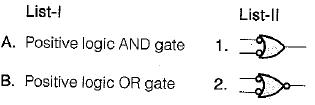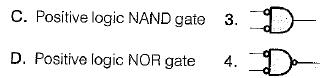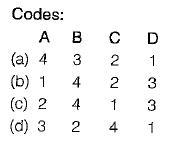Solution:
• Positive logic AND gate is equivalent to bubbled NOR gate.
• Positive logic OR gate is equivalent to bubbled NAND gate.
• Positive logic NAND gate is equivalent to bubbled OR gate.
• Positive logic NOR gate is equivalent to bubbled AND gate.
QUESTION: 2

### The minimum number of NAND gate required to implement the logic function given by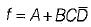is

Solution:can be implemented using 4 NAND gates as shown below: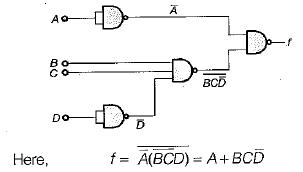QUESTION: 3

### Assertion (A): When Os and 1s are interchanged in the truth table, the positive logic AND gate becomes negative logic OR gate, and positive logic NAND gate becomes negative logic NOR gate. Reason (R): The simple method of converting the logic designation (i.e. from positive to negative logic or vice versa) is that all 0’s are replaced with 1s and all 1s with Os in the truth table.

Solution: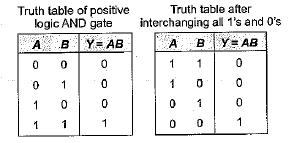Thus, we see that after interchanging all 1’s and 0’s of inputs in the truth table of positive logic AND gate, the resulting truth table obtained is the truth table of negative logic OR gate. Similarly, we can prove that if all 0’s and 1's inputs in the truth table of NAND gate are interchanged, then the gate becomes negative logic NOR gate. Hence, both assertion and reason are true and reason is the correct explanation of assertion.

QUESTION: 4

Match List-I (Logic gates) with List-ll (Applications) and select the correct answer using the codes given below the lists:

List-i
A. Exclusive OR gate
B. NOT gate
C. Exclusive NOR gate
D. NOR gate

List-ll
1. As a controlled inverter
2. As an universal gate
3. Complementation
4. Even-odd parity check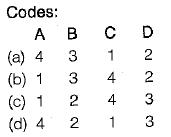Solution:
• EX-OR gate can be used as a controlled inverter as shown below: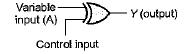For control input = 1 ,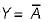For control input = 0, Y = A

• NOT gate is basically used as an inverter or for complementation purposes.
• EX-NOR gate can be used as even-odd parity checker because the output of EX- NOR gate is 1 if the number of 1’s in its inputs is even; if the number of 1’s is odd, the output is 0.
• NOR gate is an universal gate.
QUESTION: 5

Assertion (A): The EX-NOR gate can be used as a "one-bit comparator.

Reason (R): The output of the EX-NOR gate is high if the number of high inputs to it is even while the output is low if the number of high inputs to it is odd.

Solution:

An important property of the EX-NOR gate is that it can be used for bit comparison. The output of an EX-NOR gate is 1 (high) if both the inputs are similar, i.e. both are 0 or 1 (not necessarily even no. of high inputs); otherwise its output is 0 or low (not necessarily odd number of high inputs). Hence, EX-NOR gate can be used as a “one-bit comparator” . Thus, assertion is a correct statement. Reason is also a true statement but not a correct explanation of assertion.

QUESTION: 6

Consider the following logic circuit: What is the required input condition (A, B, C) to make the output X = 1, for the above logic circuit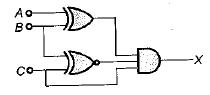Solution: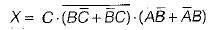Here, (0, 1, 1) gives the output X = 1

QUESTION: 7

Match List-I (Logic expression) with List-ll (Equivalent expression) and select the correct answer using the codes given below the lists: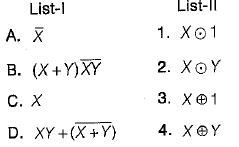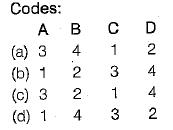Solution: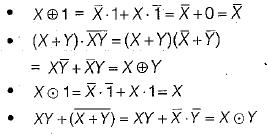QUESTION: 8

Three squares waves X, Y and Z as shown in figure below are to be ANDed. The frequency of ANDed output will be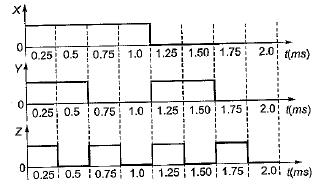Solution:

The truth table of AND gate is as shown below.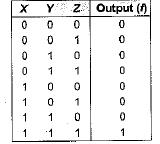The wave forms of inputs X, Y, Z and output Y are drawn below. Time period of inputs X, Y and Z are respectively 2 ms, 1 ms and 0.5 ms.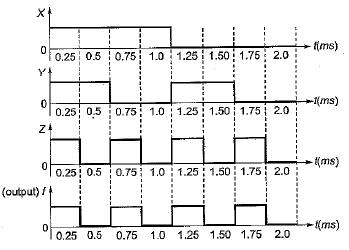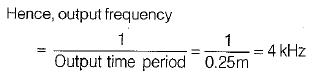QUESTION: 9

Which of the following option is not correct?

Solution:

The correct o/p waveform for given NOR gate in option (d) is shown below: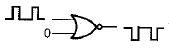Truth Table for NOR gate with one input fixed at 0 is shown below.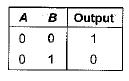QUESTION: 10

Which of the following logic gate follow both commutative and associate law?

Solution:

AND, OR, NOT are three basic gates which follow both commutative and associate law. However, the universal gates NAND and NOR don't follow associate law.